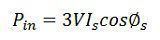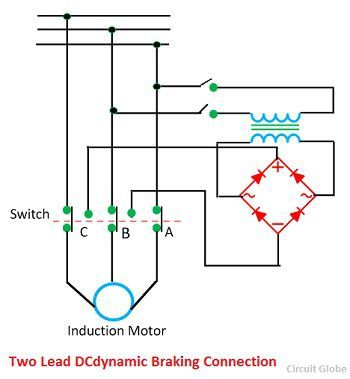# Induction Motor Braking

The braking is the process of reducing the speed of an induction motor. In braking, the motor works as a generator developing a negative torque which opposes the motion of a motor. The braking of an induction motor is mainly classified into three types. They are

1. Regenerative Braking
2. Plugging or reverse voltage braking
3. Dynamic Braking
• AC dynamic braking
• Self-dynamic braking
• DC dynamic braking
• Zero sequence braking

The braking of an induction motor is explained below in details.

## 1. Regenerative Braking

The input power of the induction motor drive is given by the formula shown belowWhere φs is the phase angle between stator phase voltage and the stator phase current Is. For motoring operation, the phase angle is always less than the 90º. If the rotor speed becomes greater than synchronous speed, then the relative speed between the rotor conductor and air gap rotating field reverse.This reverse the rotor induces emf, rotor current and component of stator current which balances the rotor ampere turns. When the φs is greater than the 90º, then the power flow to reverse and gives the regenerative braking. The magnetising current produced the air gap flux.

The nature of the speed torque curve is shown in the figure above. When the supply frequency is fixed, the regenerative braking is possible only for speeds greater than synchronous speed. With a variable frequency speed, it cannot be obtained for speed below synchronous speed.

The main advantage of regenerative braking is that the generated power is fully used. And the main drawback is that when fed from a constant frequency source the motor can not employ below synchronous speed.

## 2. Plugging

When the phase sequence of supply of the motor running at speed is reversed by interchanging the connection of any two phases of the stator on the supply terminal, operation change from motoring to plugging as shown in the figure below. Plugging is the extension of motoring characteristic for a negative phase sequence from quadrant third to second. The reversal of phase sequence reverses the direction of a rotating field.## 3. Dynamic Braking

•  AC Dynamic Braking – The dynamic braking is obtained when the motor is run on the single phase supply by disconnecting the one phase from the source and either leaving it open or connecting it with another phase. The two connections are respectively known as two and three lead connection.When connected to a one phase supply the motor can be considered as to be fed by positive and negative sequence three phase set of voltage. The total torque produced by the machine is the sum of torque due to positive and negative sequence voltage. When the rotor has high resistance, then the net torque is negative, and the braking operation is obtained.

Assume the phase A of the star connected motor is open circuited. Then the current flow through the phase A becomes zero, i.e., Ia = 0 and current through the other two phases is IB = – IC.

The positive and negative sequence component Ip and In are represented by the equation.Where α = ej20°

•  Self Excited Braking Using Capacitor – In this method the three capacitors are permanently connected to the motor. The value of the capacitor is so chosen that when disconnecting from the line, the motor works as a self-excited induction generator. The braking connection and self-excitation process is shown in the figure below.The curve A is the no load magnetisation curve and line B represent the current through the capacitor. E is the stator induced voltage per phase of the line. The capacitor supplies the necessary reactive current for excitation.

•  DC  Dynamic Braking – In this method, the stator of induction is connected across the DC supply. The method for getting DC supply with the help of a diode bridge is shown in the figure below.The direct current flow through the stator produces a stationary magnetic field, and the motion of the rotor in this field produces induces voltage in the stationary windings. The machine therefore works as a generator and the generated energy is dissipated in the rotor circuit resistance, thus giving the dynamic winding.•  Zero Sequence Braking – In this braking, the three phases of the stator are connected in series across either a single AC or DC source. Such type of connection is known as zero sequence connection because the current in all the three phases is co-phase. The nature of speed-torque curve for AC and DC supply is shown in the figure below.With the AC supply, the braking could be used only up to one-third of synchronous speed. The braking torque produces by this connection are considerable larger than motoring. With DC supply braking is available the entire speed range and the braking are essential a dynamic braking as all the generated energy is wasted into rotor resistance.